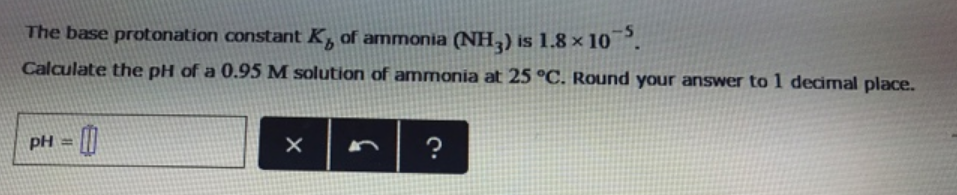Chemistry Practice Problems Weak Bases Practice Problems Solution: The base protonation constant Kb of ammonia (NH3) ...

🤓 Based on our data, we think this question is relevant for Professor White's class at OHIO.

# Solution: The base protonation constant Kb of ammonia (NH3) is 1.8 x 10 -5. Calculate the pH of a 0.95 M solution of ammonia at 25 °C. Round your answer to 1 decimal place.

###### Problem

The base protonation constant Kb of ammonia (NH3) is 1.8 x 10 -5. Calculate the pH of a 0.95 M solution of ammonia at 25 °C. Round your answer to 1 decimal place.View Complete Written Solution

Weak Bases

Weak Bases

#### Q. Determine the pH of each of the following solutions. 0.16 M NaC2H3O2. Express your answer to two decimal places.

Solved • Tue Apr 24 2018 14:27:02 GMT-0400 (EDT)

Weak Bases

#### Q. A 0.100 M solution of ethylamine (C2H5NH2) has a pH of 11.87. Calculate the Kb for ethylamine.

Solved • Tue Apr 03 2018 17:39:43 GMT-0400 (EDT)

Weak Bases

#### Q. A 25.0-mL sample of 0.125 M pyridine is titrated with 0.100 MHCl. Calculate the pH at 0 mL of added acid. Express your answer using two decimal places...

Solved • Fri Mar 30 2018 12:14:38 GMT-0400 (EDT)

Weak Bases

#### Q. Find the pH of a 0.250 M solution of NaC2H3O2. (The Ka value of HC2H3O2 is 1.80×10-5).

Solved • Tue Mar 27 2018 14:00:55 GMT-0400 (EDT)